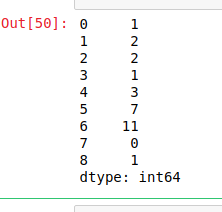# Python | Pandas Series.combine()

Python is a great language for doing data analysis, primarily because of the fantastic ecosystem of data-centric Python packages. Pandas is one of those packages and makes importing and analyzing data much easier.

Pandas `Series.combine()` is a series mathematical operation method. This is used to combine two series into one. The shape of output series is same as the caller series. The elements are decided by a function passed as parameter to `combine()` method. The shape of both series has to be same otherwise it will throw an error.

Syntax: Series.combine(other, func, fill_value=nan)

Parameters:
other: other series or list type to be combined with caller series
func: Function passed as parameter which will decide from which series the element should be put at that index
fill_value: integer value of level in case of multi index

Return: Combined series with same shape as caller series

Example #1:

In this example, two lists are made and converted into pandas series using .Series() method. A function is made using lambda which checks which values is smaller in both series and returns whichever is the smaller.

 `# importing pandas module ` `import` `pandas as pd ` ` `  `# creating first series ` `first ``=``[``1``, ``2``, ``5``, ``6``, ``3``, ``7``, ``11``, ``0``, ``4``] ` ` `  `# creating second series ` `second ``=``[``5``, ``3``, ``2``, ``1``, ``3``, ``9``, ``21``, ``3``, ``1``] ` ` `  `# making series ` `first ``=` `pd.Series(first) ` ` `  `# making seriesa ` `second ``=` `pd.Series(second) ` ` `  `# calling .combine() method ` `result ``=` `first.combine(second, (``lambda` `x1, x2: x1 ``if` `x1 < x2 ``else` `x2)) ` ` `  `# display ` `result `

Output:
As shown in the output image, the returned series is having smaller values from both series.Example #2:

In this example, Null values are passed too using `Numpy.nan` method. Since series contains null values, 5 is passed to fill_value parameter to replace null values by 5. A lambda function is passed which will compare values in both series and will return the greater one.

 `# importing pandas module ` `import` `pandas as pd ` ` `  `# importing numpy module ` `import` `numpy as np ` ` `  `# creating first series ` `first ``=``[``1``, ``2``, np.nan, ``5``, ``6``, ``3``, np.nan, ``7``, ``11``, ``0``, ``4``, ``8``] ` ` `  `# creating second series ` `second ``=``[``5``, ``3``, ``2``, np.nan, ``1``, ``3``, ``9``, ``21``, ``3``, np.nan, ``1``, np.nan] ` ` `  `# making series ` `first ``=` `pd.Series(first) ` ` `  `# making seriesa ` `second ``=` `pd.Series(second) ` ` `  `# calling .combine() method ` `result ``=` `first.combine(second, func ``=``(``lambda` `x1, x2: x1 ``if` `x1 > x2 ``else` `x2), fill_value ``=` `5``) ` ` `  `# display ` `result `

Output:
As shown in the output, the NaN values in the series were replaced by 5 before combining the series.

```
0      5.0
1      3.0
2      2.0
3      5.0
4      6.0
5      3.0
6      9.0
7     21.0
8     11.0
9      5.0
10     4.0
11     8.0
dtype: float64```

My Personal Notes arrow_drop_upCheck out this Author's contributed articles.

If you like GeeksforGeeks and would like to contribute, you can also write an article using contribute.geeksforgeeks.org or mail your article to contribute@geeksforgeeks.org. See your article appearing on the GeeksforGeeks main page and help other Geeks.

Please Improve this article if you find anything incorrect by clicking on the "Improve Article" button below.

Improved By : Akanksha_Rai

Article Tags :

1

Please write to us at contribute@geeksforgeeks.org to report any issue with the above content.